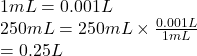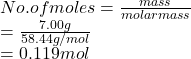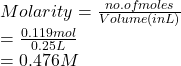## A 7.00 g sample of NaCl was dissolved in enough water to give 250 mL solution. What is the molarity of the solution?

Question

A 7.00 g sample of NaCl was dissolved in enough water to give 250 mL solution.
What is the molarity of the solution?

in progress 0
2 weeks 2021-08-29T19:13:25+00:00 1 Answers 0 views 0

1. Answer: The molarity of given solution is 0.476 M.

Explanation:

Given : mass of sample (solute) = 7.0 g

Volume of solution (solvent) = 250 mL

Convert mL into L as follows.Molar mass of NaCl is 58.44 g/mol. Hence, moles of NaCl are calculated as follows.Molarity is the number of moles of a substance present in one liter of solution or solvent.

So, molarity of given solution is calculated as follows.Thus, we can conclude that molarity of given solution is 0.476 M.